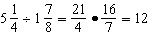Name:    Quiz 2.5-2.8

Multiple Choice
Identify the choice that best completes the statement or answers the question.

Evaluate the expression when a =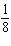and b =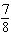.

1.

a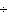b
 a.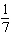c.b.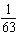d.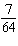Evaluate the expression.

2.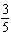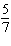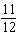a.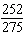c.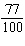b.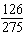d.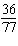3.

5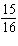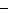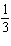a.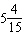c.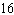b.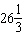d.Write the reciprocal of the number.

4.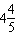a.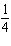c.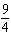b.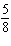d.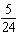5.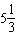4
 a.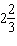c.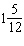b.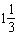d.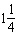6.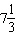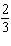a.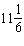c. 11 b. 12 d.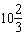7.

How many minutes does it take to put 45 gallons of water in the tub at a rate of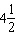gallons per minute?

 a.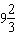min c.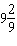min b.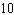min d.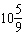min

Evaluate the expression when x =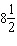and y =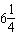.

8.

y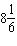a.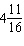c.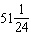b.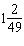d.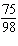Evaluate the expression.

9.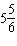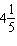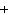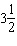a.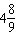c.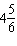b.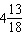d.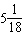Write the decimal as an improper fraction in simplest form.

10.

7.25
 a.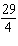c.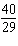b.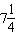d.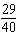Write the fraction as a decimal.

11.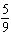a.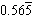c. 0.56 b. 0.55 d.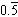Numeric Response

1.

Your batting average for a baseball season is. Write your batting average as a decimal.